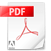## Finite strain and Birch–Murnaghan Equations of State

### Abstract

The Eulerian finite strain of an elastically isotropic body is defined using the expansion of squared length and the post-compression state as reference. The key to deriving second-, third- and fourth-order Birch–Murnaghan equations-of-state (EOSs) is not requiring a differential to describe the dimensions of a body owing to isotropic, uniform, and finite change in length and, therefore, volume. Truncation of higher orders of finite strain to express the Helmholtz free energy is not equal to ignoring higher-order pressure derivatives of the bulk modulus as zero. To better understand the Eulerian scheme, finite strain is defined by taking the pre-compressed state as the reference, and EOSs are derived in both the Lagrangian and Eulerian schemes. In the Lagrangian scheme, pressure increases less significantly upon compression than the Eulerian scheme. Different Eulerian strains are defined by the expansion of linear and cubed length, and the first- and third-power Eulerian EOSs are derived in these schemes. Fitting analysis of pressure-scale-free data using these equations indicates that the Lagrangian scheme is inappropriate to describe P-V-T relations of MgO, whereas three Eulerian EOSs, including the Birch–Murnaghan EOS, have equivalent significance.

Plain explanations are given in the lecture page about equations of state in Tomo Katsura's Website.

In more detail,

Full discussion is of course given in the publication

Hugoniot curves of MgO reproduced by the various EOSs using pressure scale-free data following Tange et al. . The dashed light-red, thin-solid red, dotted dark-red, and solid blue curves are obtained from the first-, second-, and fourth- Eulerian and second-power Lagrangian EOSs, respectively. The light-gray square and dark-gray circle are experimental data obtained by Marsh  and Duffy and Ahrens , respectively.

Since  the equations of state based on different definitions of the Eulerian finite strain provide essentially identical compressional curves, the definition of the Eulerian finite strain is somewhat arbitrary. This implies that the Birch-Murnaghan equation of state does not have significant physical meaning.A Simple Derivation of the Birch–Murnagh